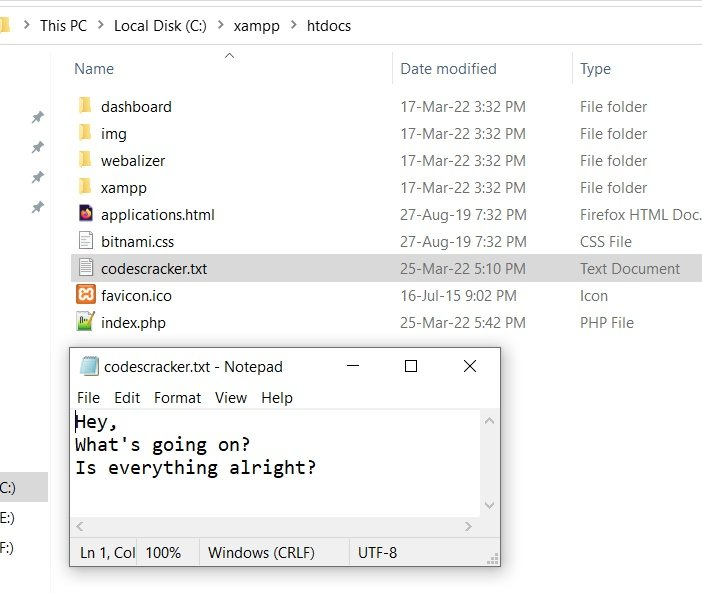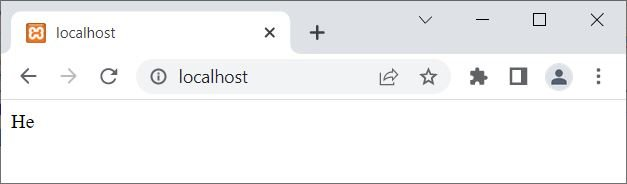# PHP fgets(): Get a Single Line from a File

The PHP fgets() function is used when we need to read a file line by line. For example:

```<?php
\$fp = fopen("codescracker.txt", "r");
echo fgets(\$fp);
fclose(\$fp);
?>```

The output produced by the above PHP example on the fgets() function is:The text "Hey," as shown in the output shown in the above snapshot, is the text of the first line of the file named codescracker.txt. Here is the snapshot of the opened file, available in the current directory:Note: The fopen() function opens a file.

Note: The fclose() function closes a file.

To get all lines using fgets(), you need to go through the whole content of the file using the feof() function. Here is an example:

```<?php
\$fp = fopen("codescracker.txt", "r");
if(\$fp)
{
while(!feof(\$fp))
{
\$line = fgets(\$fp);
echo \$line;
echo "<br>";
}
fclose(\$fp);
}
else
echo "<p>Unable to open the file!</p>";
?>```

Now the output should be:Note: The feof() function checks whether the file pointer has been reached at the end of the file.

## PHP fgets() Syntax

The syntax of the fgets() function in PHP is:

`fgets(filePointer, size)`

The filePointer parameter is required, whereas the size parameter is optional.

Note: The size parameter is used when we need to fetch up to a particular number of bytes. For example:

```<?php
\$fp = fopen("codescracker.txt", "r");
if(\$fp)
{
\$content = fgets(\$fp, 3);
echo \$content;
fclose(\$fp);
}
else
echo "<p>Unable to open the file!</p>";
?>```

The output produced by the above PHP example on the fgets() function with the size parameter is:Note: The reading of the file stops when a new line occurs. Therefore, since the first line of the codescracker.txt file contains Hey,. That can be read using fgets(\$fp, 5). But if you increase the size, then in that case too, you will get the same output, which will be the content of the first line.

PHP Online Test

« Previous Tutorial Next Tutorial »